Most Affordable JEE | NEET | 8,9,10 Preparation by Kota's Top IITian Doctor Faculties

# NCERT Solutions for Class 9 Maths chapter 7 Exercise 7.1 - Triangles

Class 9Hey, are you a class 9 Student and Looking for Ways to Download NCERT Solutions for Class 9 Maths chapter 7 Exercise 7.1? If Yes then you are at the right place.

Here we have listed Class 9 maths chapter 7 exercise 7.1 solutions in PDF that is prepared by Kota’s top IITian’s Faculties by keeping Simplicity in mind.

If you want to score high in your class 9 Maths Exam then it is very important for you to have a good knowledge of all the important topics, so to learn and practice those topics you can use eSaral NCERT Solutions.

So, without wasting more time Let’s start.

### Download The PDF of NCERT Solutions for Class 9 Maths chapter 7 Exercise 7.1 "Triangles"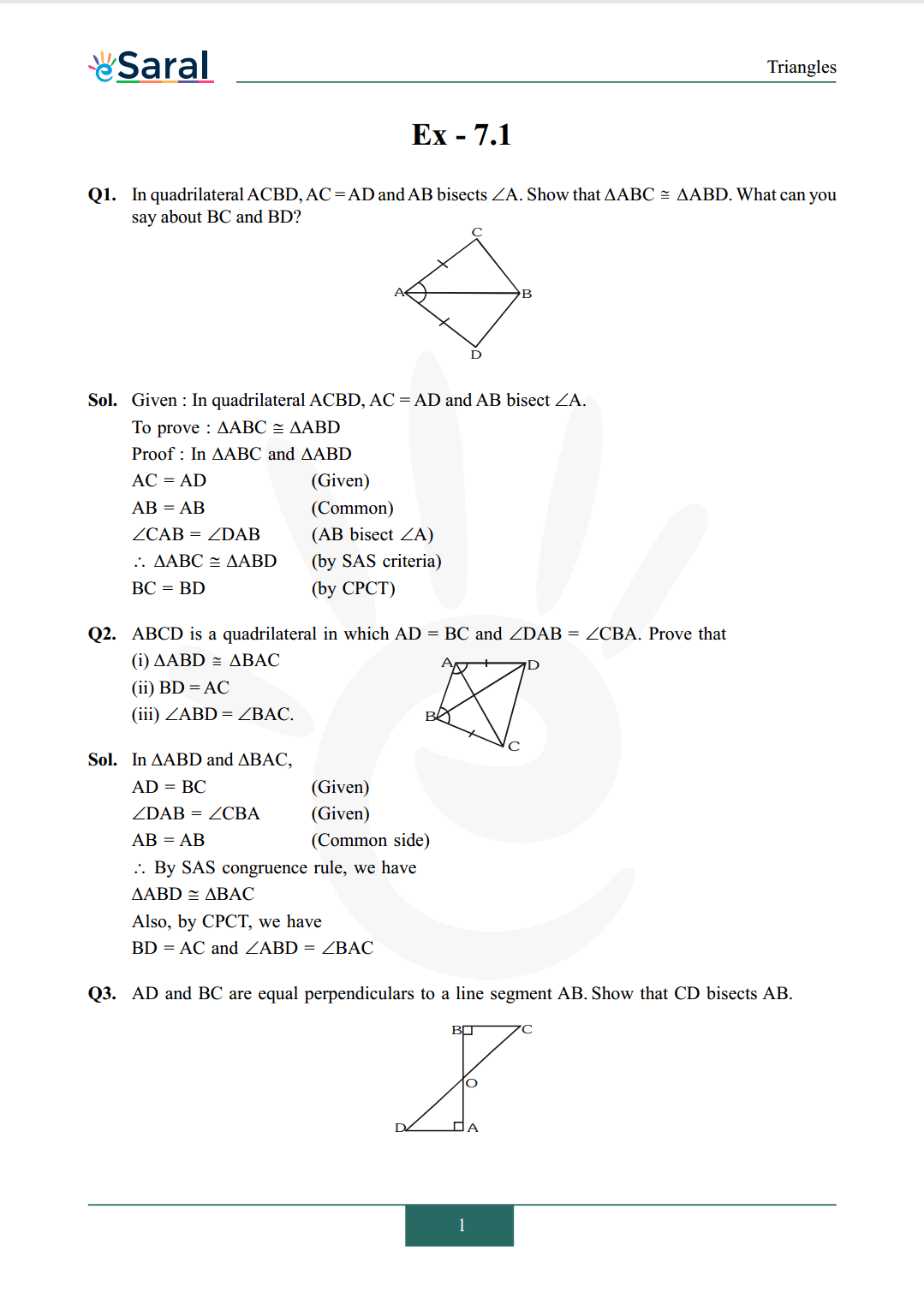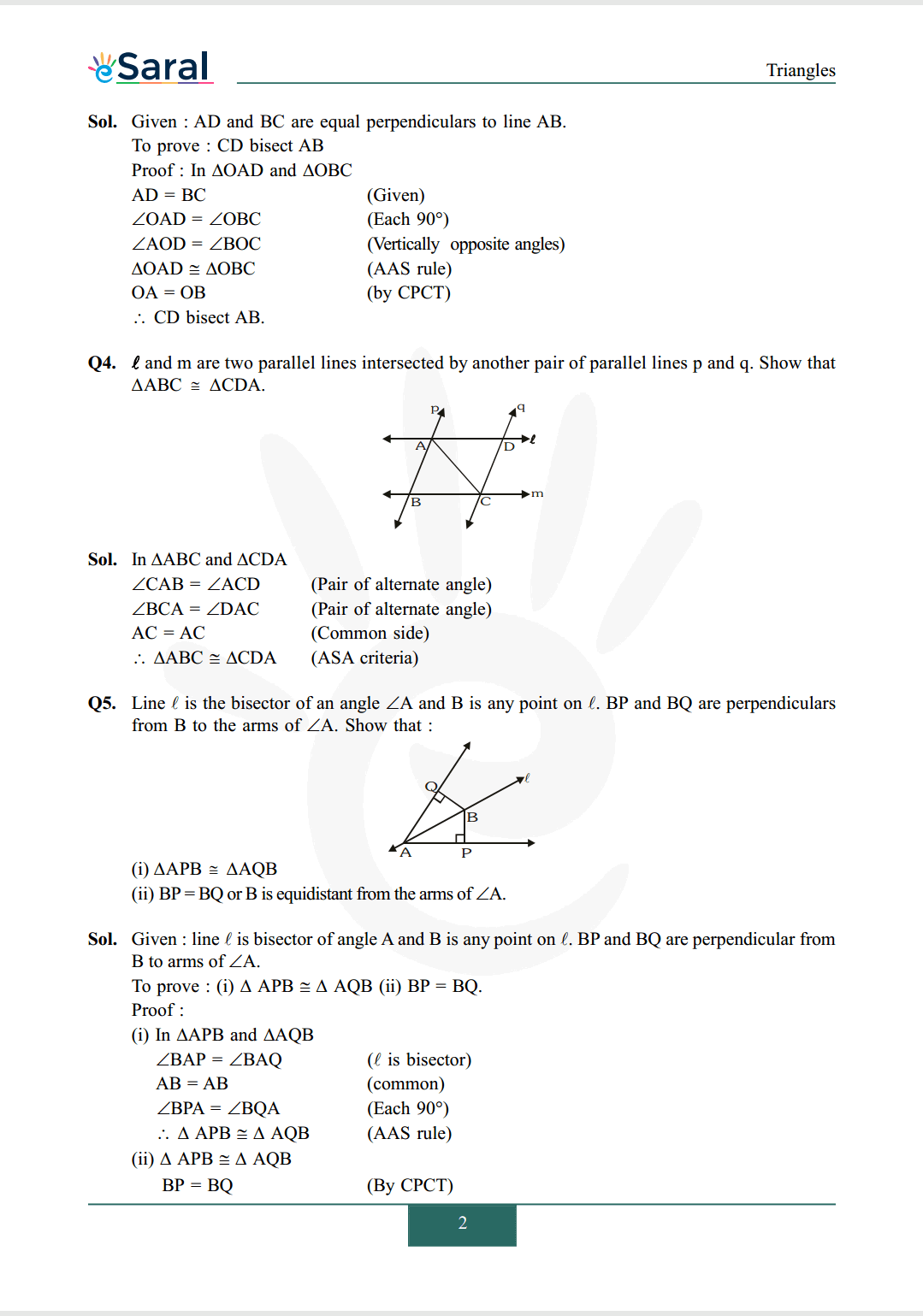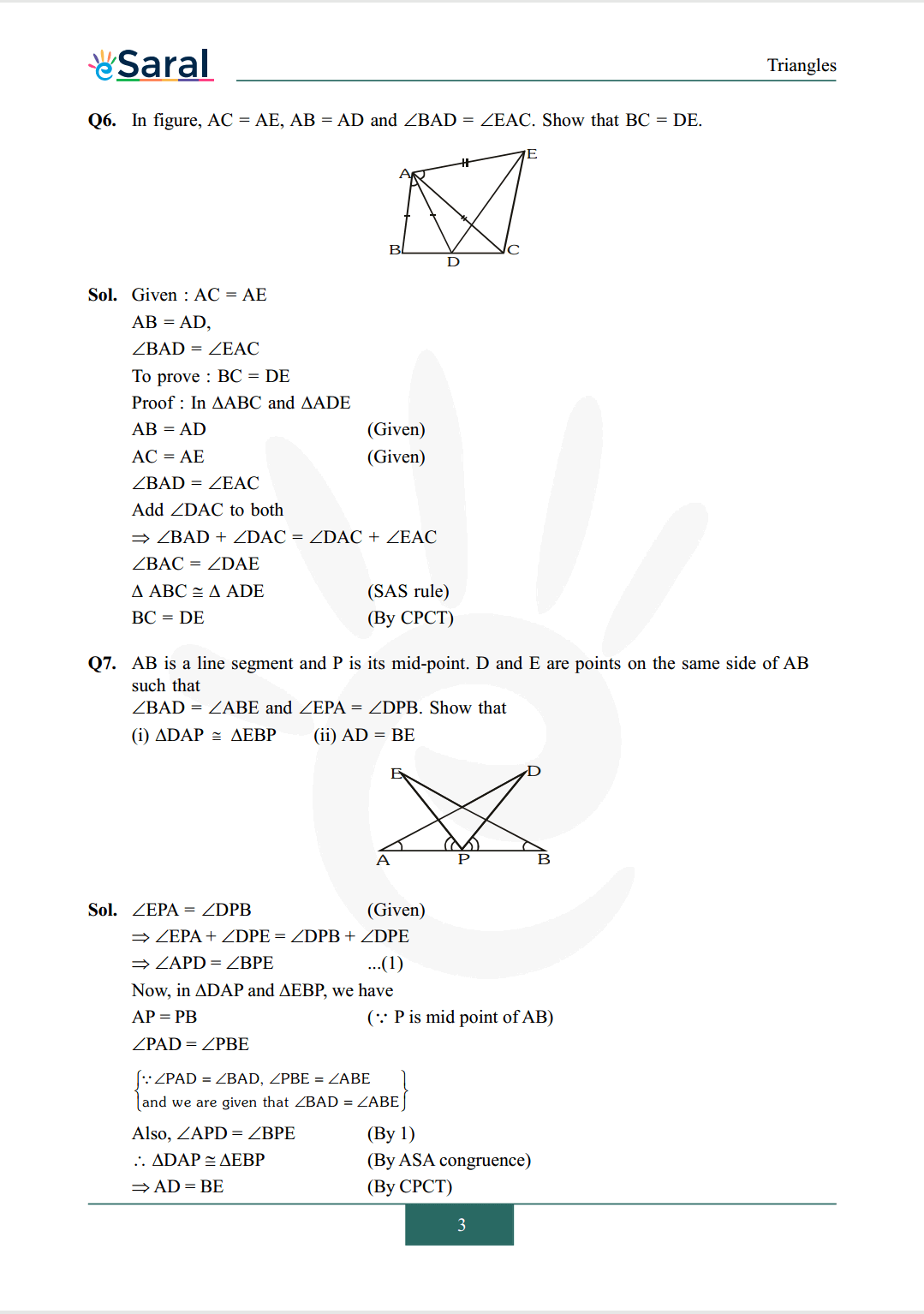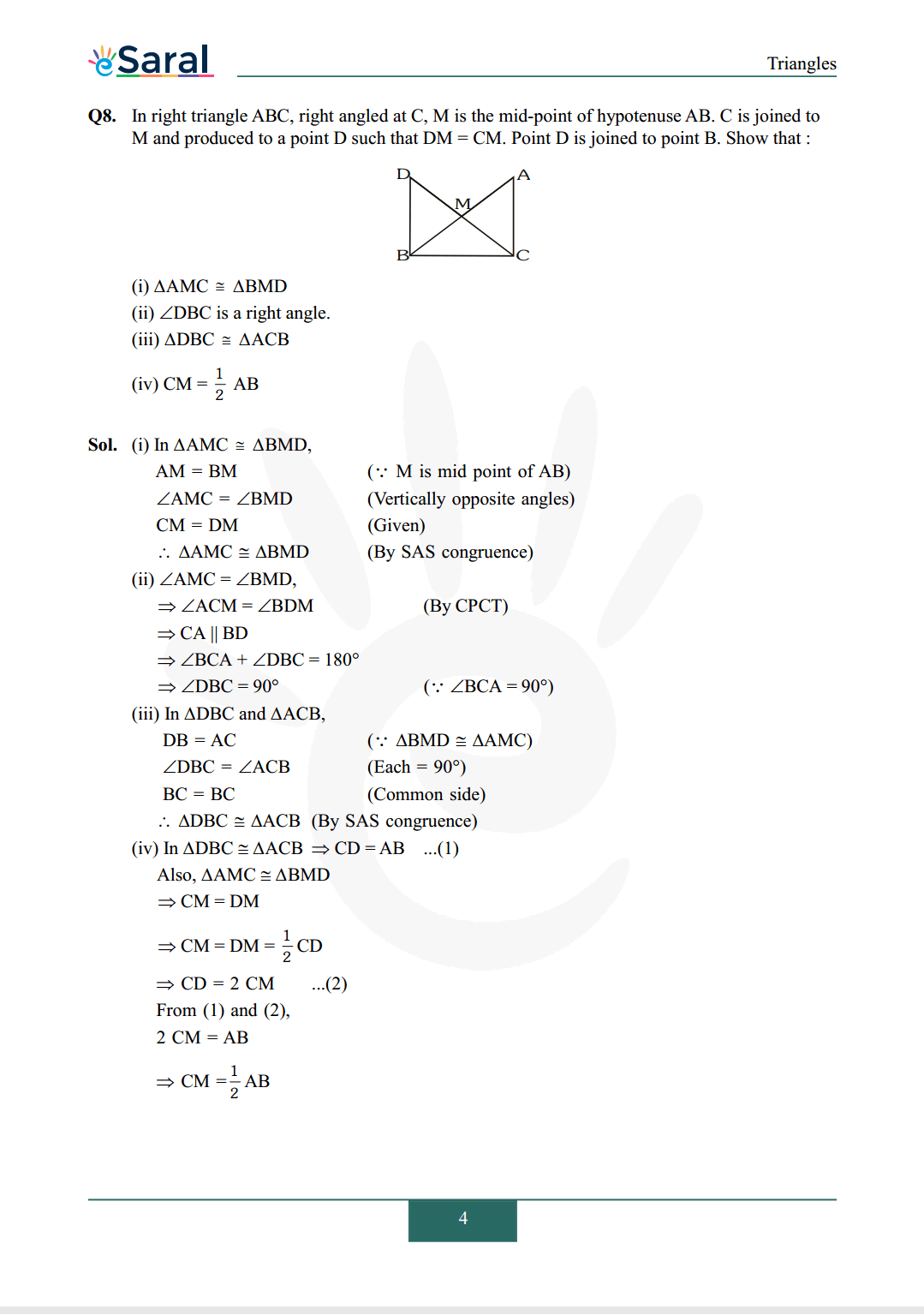#### All Questions of Chapter 7 Exercise 7.1

Once you complete the chapter 7 then you can revise Ex. 7.1 by solving following questions

Q1.In quadrilateral $\mathrm{ACBD}, \mathrm{AC}=\mathrm{AD}$ and $\mathrm{AB}$ bisects $\angle \mathrm{A}$. Show that $\triangle \mathrm{ABC} \cong \triangle \mathrm{ABD}$. What can you say about $\mathrm{BC}$ and $\mathrm{BD}$ ?Q2. $\mathrm{ABCD}$ is a quadrilateral in which $\mathrm{AD}=\mathrm{BC}$ and $\angle \mathrm{DAB}=\angle \mathrm{CBA}$. Prove that
(i) $\Delta \mathrm{ABD} \cong \Delta \mathrm{BAC}$
(ii) $\mathrm{BD}=\mathrm{AC}$
(iii) $\angle \mathrm{ABD}=\angle \mathrm{BAC}$.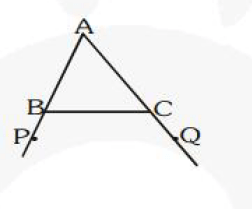Q3. AD and BC are equal perpendiculars to a line segment AB. Show that CD bisects AB.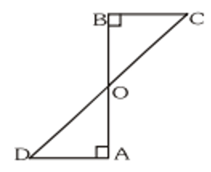Q4. $\ell$ and $\mathrm{m}$ are two parallel lines intersected by another pair of parallel lines $\mathrm{p}$ and $\mathrm{q}$. Show that $\Delta \mathrm{ABC} \cong \Delta \mathrm{CDA}$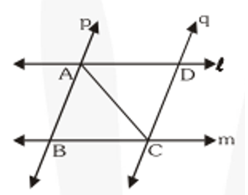Q5. Line $\ell$ is the bisector of an angle $\angle \mathrm{A}$ and $\mathrm{B}$ is any point on $\ell$. BP and $\mathrm{BQ}$ are perpendiculars from $\mathrm{B}$ to the arms of $\angle \mathrm{A}$. Show that :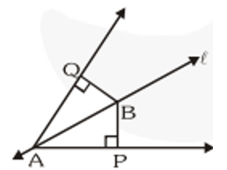(i) $\triangle \mathrm{APB} \cong \triangle \mathrm{AQB}$
(ii) $\mathrm{BP}=\mathrm{BQ}$ or $\mathrm{B}$ is equidistant from the arms of $\angle \mathrm{A}$.

Q6. In figure, $\mathrm{AC}=\mathrm{AE}, \mathrm{AB}=\mathrm{AD}$ and $\angle \mathrm{BAD}=\angle \mathrm{EAC}$. Show that $\mathrm{BC}=\mathrm{DE}$.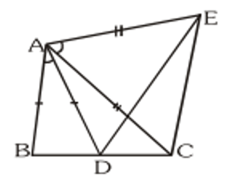Q7. $\mathrm{AB}$ is a line segment and $\mathrm{P}$ is its mid-point. $\mathrm{D}$ and $\mathrm{E}$ are points on the same side of $\mathrm{AB}$ such that $\angle \mathrm{BAD}=\angle \mathrm{ABE}$ and $\angle \mathrm{EPA}=\angle \mathrm{DPB}$. Show that
(i) $\Delta \mathrm{DAP} \cong \Delta \mathrm{EBP}$
(ii) $\mathrm{AD}=\mathrm{BE}$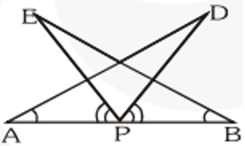Q8. In right triangle $\mathrm{ABC}$, right angled at $\mathrm{C}, \mathrm{M}$ is the mid-point of hypotenuse $\mathrm{AB} . \mathrm{C}$ is joined to $\mathrm{M}$ and produced to a point
$\mathrm{D}$ such that $\mathrm{DM}=\mathrm{CM}$. Point $\mathrm{D}$ is joined to point $\mathrm{B}$. Show that :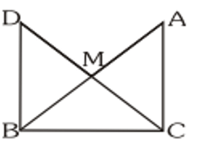(i) $\triangle \mathrm{AMC} \cong \Delta \mathrm{BMD}$
(ii) $\angle \mathrm{DBC}$ is a right angle.
(iii) $\triangle \mathrm{DBC} \cong \triangle \mathrm{ACB}$
(iv) $\mathrm{CM}=\frac{1}{2} \mathrm{AB}$